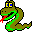#Boost C++ Libraries

...one of the most highly regarded and expertly designed C++ library projects in the world.

This is the documentation for an old version of Boost. Click here to view this page for the latest version.#strong_components

```// named parameter version
template <class Graph, class ComponentMap, class P, class T, class R>
typename property_traits<ComponentMap>::value_type
strong_components(Graph& g, ComponentMap comp,
const bgl_named_params<P, T, R>& params = all defaults)

// there is not a non-named parameter version of this function
```

The strong_components() functions compute the strongly connected components of a directed graph using Tarjan's algorithm based on DFS .

The output of the algorithm is recorded in the component property map comp, which will contain numbers giving the component ID assigned to each vertex. The number of components is the return value of the function.

### Definitions

A strongly connected component of a directed graph G=(V,E) is a maximal set of vertices U which is in V such that for every pair of vertices u and v in U, we have both a path from u to v and path from v to u. That is to say that u and v are reachable from each other.

### Parameters

IN: const Graph& g
A directed graph. The graph type must be a model of Vertex List Graph and Incidence Graph.
Python: The parameter is named graph.
OUT: ComponentMap c
The algorithm computes how many connected components are in the graph, and assigning each component an integer label. The algorithm then records which component each vertex in the graph belongs to by recording the component number in the component property map. The ComponentMap type must be a model of Writable Property Map. The value type shouch be an integer type, preferably the same as the vertices_size_type of the graph. The key type must be the graph's vertex descriptor type.
Python: Must be an vertex_int_map for the graph.
Python default: graph.get_vertex_int_map("component")

### Named Parameters

UTIL: root_map(RootMap r_map)
This is used by the algorithm to record the candidate root vertex for each vertex. By the end of the algorithm, there is a single root vertex for each component and get(r_map, v) returns the root vertex for whichever component vertex v is a member. The RootMap must be a Read/Write Property Map, where the key type and the value type are the vertex descriptor type of the graph.
Default: an iterator_property_map created from a std::vector of vertex descriptors of size num_vertices(g) and using the i_map for the index map.
Python: Unsupported parameter.
UTIL: discover_time_map(TimeMap t_map)
This is used by the algorithm to keep track of the DFS ordering of the vertices. The TimeMap must be a model of Read/Write Property Map and its value type must be an integer type. The key type must be the vertex descriptor type of the graph.
Default:an iterator_property_map created from a std::vector of integers with size num_vertices(g) and using the i_map for the index map.
Python: Unsupported parameter.
UTIL: color_map(ColorMap c_map)
This is used by the algorithm to keep track of its progress through the graph. The type ColorMap must be a model of Read/Write Property Map and its key type must be the graph's vertex descriptor type and the value type of the color map must model ColorValue.
Default: an iterator_property_map created from a std::vector of default_color_type of size num_vertices(g) and using the i_map for the index map.
Python: Unsupported parameter.
IN: vertex_index_map(VertexIndexMap i_map)
This maps each vertex to an integer in the range [0, num_vertices(g)). This parameter is only necessary when a default is used for one of the other named parameters. The type VertexIndexMap must be a model of Readable Property Map. The value type of the map must be an integer type. The vertex descriptor type of the graph needs to be usable as the key type of the map.
Default: get(vertex_index, g) Note: if you use this default, make sure your graph has an internal vertex_index property. For example, adjacenty_list with VertexList=listS does not have an internal vertex_index property.
Python: Unsupported parameter.

### Complexity

The time complexity for the strongly connected components algorithm is O(V + E).

### See Also

connected_components() and incremental_components()

### Example

 Copyright © 2000-2001 Jeremy Siek, Indiana University (jsiek@osl.iu.edu)